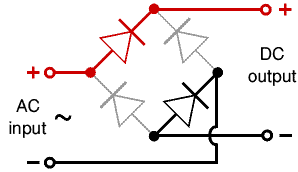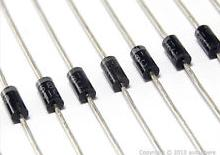# Bridge Rectifier

For most alternative energy applications, we require a direct current (DC) voltage to be generated – for example to charge a bank of batteries. However wind turbines and wave power generators create an alternating current (AC) voltage. This is where the Bridge Rectifier comes in. The AC voltage generated is passed through a circuit of four diodes arranged as shown below and emerges converted into the desired DC output.Diodes only allow electricity to flow in one direction, but there is a small amount of voltage lost as the current passes through a diode of 0.7V (silicon diodes) called the forward voltage drop. To halve the forward voltage drop, a Schottky diode or germanium diode can be used. The forward voltage drop is particularly significant in very low voltage (e.g. <12 Volt) applications as 0.7*2=1.4V is dropped in a silicon bridge rectifier (x2 because the current passes through two diodes on its way through the bridge rectifier).

If the diode is wired in the reverse direction then no current [actually a very tiny current] flows across the diode. However, if the voltage is too high and goes over the diode’s maximum reverse voltage(detailed in the specification sheet for any diode), the diode will breakdown and fail.If you would like to make your own bridge rectifier then the 1N4001 diode is perfect for most low voltage circuits where the current is less than 1A. The 1N5401 diode is often used where the maximum current is up to 3A. These diodes are extremely cheap and available everywhere. Click here to buy Schottky diodes.